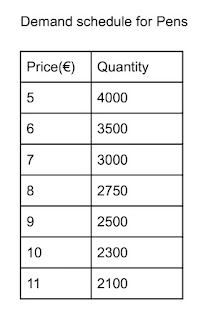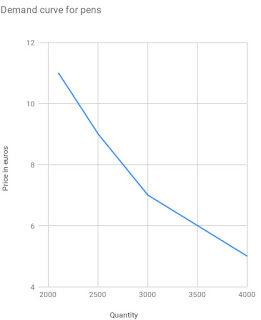# DEMAND, DEMAND SCHEDULE, DEMAND CURVE AND DEMAND FUNCTION

0
Demand refers to the quantity of a product that a consumer is willing and able to buy at a given price. From this definition, you can pinpoint two words, "willingness" and "ability to buy".

Willingness to pay implies the desire for a good.

The ability to buy implies consumers should be able to pay for what he is willing to buy. The ability to pay is usually based on consumer income. If any of the two factors are not present, then, there is no effective demand.

Willingness and ability to pay differentiate demand from want because demand is want or need backed by the willingness and ability to pay.

For example, John may be willing to buy a pen but be unable to pay the price; Daniel may be able to buy a pen but be unwilling to do so.

Neither John nor Daniel has an effective demand for the pen. John and Daniel can be said to merely want a pen but do not demand it.

What a consumer pays for particular goods or services is known as price. A specific unit of a good that individuals are willing and able to buy is called the quantity demanded.

When the price of goods increases, the quantity demanded decreases; when the price of goods decreases, the quantity increases.

Economists called this "the law of demand". The law of demand holds all other factors constant.

The inverse relationship between price and quantity demanded may be represented on a table.

Economists call this table demand schedule. A demand schedule shows the quantity demanded at different prices.
Here is an exampleThe above information can also be represented on a demand curve

A demand curve is a graphical representation of the quantity demanded at each price.

It is essentially a graphical view of the price and quantity pairing listed in the demand schedule.

A typical demand curve is downward-sloping, reflecting the inverse relationship between quantity demand and the price of the goods. Here is a demand curve.## Differences Between Change In Demand And Change In Quantity Demand.

In economics, the change in quantity demanded is not the same as the change in demand. Thus, it is necessary that we distinguished between these two phenomena.

As you just saw above, when own-price changes, the quantity demanded also changes, this is called a change in quantity demanded or movement along the same demand curve.

Changes in quantity demanded are usually attributed to changes in good own price.

The rate at which quantity demanded changes concerning price changes can be measured by the price elasticity of demand.

Change in demand, on the other hand, is caused by non-price. What are these other factors that affect demand? and how do they affect demand? this and more are answered below:

### Income

For demand to be effective, it must be characterized by the ability to pay.

Your ability to pay invariably hinges on your income. To a large extent, this is determined by your budget constraints

Generally, there is a direct relationship between income and quantity demanded. This means an increase in income will cause an outward shift in demand (to the right).

Goods and services characterized by this kind of relationship are called Normal goods.  Houses and cars are examples of normal goods.

Though most goods and services are normal goods, there are exceptions.

These exceptions are characterized by a negative relationship, with less being demanded as income rises, and more demanded as income decreases. Economists called these goods inferior goods

An example of such goods is secondhand cars. With a lower income, the consumer will have no choice but to settle for such a car. As his income increases, he would likely buy a new car instead of a secondhand car.

However, for some goods, a change in income does not necessarily affect their demand. These kinds of goods are called neutral goods.

### Price of related goods

Like I said before, the demand for goods is also affected by the price of related goods. Two kinds of goods can be identified here: substitute goods and complementary goods

Speaking of substitute goods. Let's assume that there is an increase in the price of Coca-cola. Consumers, who don't see any difference between Pepsi and Coca-cola would prefer to buy Pepsi.

After all, Pepsi is now cheaper than coca-cola. Thus, the demand curve for Pepsi will shift outward(to the right), and the demand curve for Coca-cola may shift inward(to the left).

One thing you should note about substitute goods is that the change in demand for the two goods largely depends on the degree of substitutability of the two goods concerned. The higher the substitutability, the more likely the goods can be substituted

Complimentary goods, on the other hand, enhance the consumer satisfaction derived from each one.

A consumer, for example, who bought a notebook would also buy a pen or pencil. After all, he can't write in a notebook without a pen or pencil.

This means that when the price of the notebook increases, consumers may not buy a notebook or a pen/pencil.

In other words, when there is an increase in the price of a notebook, the demand curve for pen/pencil will shift inward.

### Changes in Consumer taste or attitude

Just as the ability to pay depends on the consumer's income, the willingness to pay also hinges on the consumer's taste.

Every consumer has a unique taste or preference. Hence, the popular line "there is no accounting for taste". This consumer taste can be measured on an indifferent curve.

Let's take, for example, consumers who love printed books. They will always buy printed books regardless of the availability of an electronic book (e-book).

If for some reason,(like e-books durability), these consumers choose to start buying e-books rather than printed books, then the demand curve of the e-book will shift to the right while the demand curve of printed books would shift to the left.

Demand also depends invariably on the number of buyers in the market. So, more buyers, higher demand; Fewer buyers, lesser demand.

Factors such as higher birth rates, higher death rates, and migration would influence the number of buyers in the market.

### Consumer expectations about future prices or availability

Many factors affect demand. One such factor is the circumstances of the economy, e.g, emergencies. An instance is when the news of Covid-19 broke out.

Even at a high price, many consumers bought face masks.

Many of these consumers wouldn't have bought such face masks (even if they were sold for just 5 cents). The news of covid-19 shifts the demand curve of the face mask outward.

Another factor that could affect the demand for goods is the "expectation of future price rise".

When a consumer learns that the price of rice could increase or rice would be scarce, he will quickly stock up on rice so as avoid buying it at a higher price. This action will shift the present demand curve for rice outward.

When the above factors affect, it is called a shift in the demand curve/changes in demand. The accompanying diagram shows a shift in the demand curve.

When the demand curve shifts inward ( from point $D_2$ to $D_0$), it is called a decrease in demand

Conversely, an outward shift of the demand curve( from point $D_0$ to $D_2$) is called an increase in demand.

Therefore, a change in quantity demanded is an expansion or contraction in demand caused by the good's price while the change in demand is an increase or decrease in demand caused by other non-price factors while keeping the good own-price constant.

Related post
All the factors that influence demand (both price and non-price) can be represented in a demand function

A demand function expresses the demand for goods and services as a function of price and other determinants of demand

$Q_x^d=f(p_x, y, p_y$, ...)

Where,
$Q_x^d$ represents the quantity of demanded goods x
$p_x$ represent the price of good a
y represents the income of consumers
$p_y$ represents the price of related goods

Example
A consumer weekly demand for the pen is given by the demand function:
$Q_{pn}^d=18-0.5P_{pn}+0.04Y-0.3P_n$.
Where $Q_{pn}^d$ is the number of pens demanded weekly
$P_{pn}$ is the price of the pen
Y is the consumer income
$P_n$ is the price of the notebook
Assuming the price of the pen is €20, consumer income is €1000, and the price of the notebook is €10.
1) Determine the number of pens demanded weekly
2) Determine the inverse demand function given the value of y and $P_n$

Take note of the sign of the coefficient of each variable. The sign of the coefficient of consumer income is positive, indicating that the good concerned is a normal good.

The sign of the coefficient of related goods price is positive, indicating that the pen and notebook have a negative cross-price elasticity of demand and are therefore complementary goods.

1) $Q_{pn}^d=18-0.5P_{pn}+0.04Y-0.3P_n$.
Insert the value of each variable
$Q_{pn}^d=18-0.5(20)+0.04(1000)-0.3(10)$
$Q_{pn}^d=18-10+40-3$
$Q_{pn}^d=45$
The consumer weekly demand for pen consumers is 45

2)First, let's find the price-quantity relationship.

$Q_{pn}^d=18-0.5P_{pn}+0.04Y-0.3P_n$
$Q_{pn}^d=18-0.5P_{pn}+0.04(1000)-0.3(10)$
$Q_{pn}^d=18-0.5P_{pn}+40-3$
$Q_{pn}^d=18-0.5P_{pn}+37$
$Q_{pn}^d=55-0.5P_{pn}$

The inverse demand function means we should make price the subject of the function.

So we are going to do simple maths

$Q_{pn}^d=55-0.5P_{pn}$
Let's make the price subject to the formula
$0.5P_{pn}=55-Q_{pn}^d$
Divide both sides by 0.5
$\frac{\require{cancel}\bcancel{0.5}P_{pn}}{\require{cancel}\bcancel{0.5}}=\frac{55-Q_{pn}^d}{0.5}$
$P_{pn}=\frac{55-Q_{pn}^d}{0.5}$

The demand function is important for solving price discrimination and marginal revenue.

Not to mention, the interaction of demand and supply help determine the market equilibrium.

Furthermore, there are seven types of demand:  Joint demand, competitive demand, composite demand, market demand, individual demand, direct demand and indirect demand. You would get to know more about them here.

Ok, That's all for now. If you have educational questions, you can tell me in the comment box. Alternatively, you can do so in our Facebook community  or telegram community
Tags